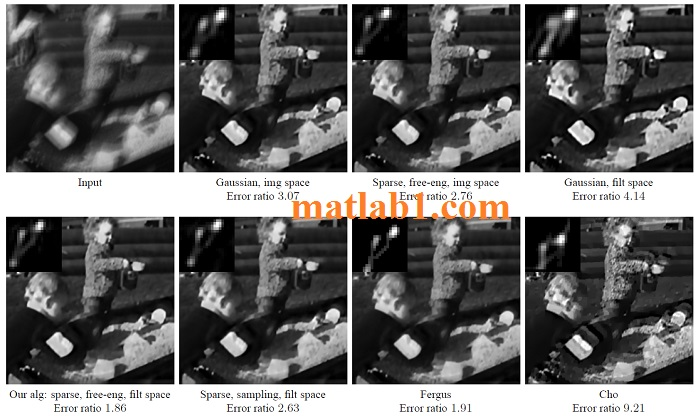# Efficient Marginal Likelihood Optimization in Blind Deconvolution

\$39

## Description

The MAPk blind deconvolution principle is significantly more robust than the MAPx,k principle. Yet, it is considered hard to implement and has not been widely exploited.
In this paper we argue that the MAPk approach can actually be optimized easily, and present simple and practical MAPk algorithms. While popular MAPx,k strategies basically alternate between latent image estimation given a kernel and kernel estimation given an image, our MAPk algorithm employs the same steps, where the only difference is that the kernel estimation accounts for the covariance around x and not only for the mean solution. While an exact estimation of
the covariance is challenging, a diagonal approximation can be computed efficiently in O(N) as the inverse diagonal of the deconvolution system.
While we have presented the basic principles of MAPk optimization, there are many more algorithmic choices to explore, such as the choice of filters, the choice of covariance approximation, and the prior model.Recovered images for two test examples. We empirically observe that deconvolution results are visually plausible when the ratio of errors between deconvolution with the estimated kernel and deconvolution with the ground truth kernel is below 3.

Robust Point Matching Revisited: A Concave Optimization Approach

DREAM OPTIMIZATION INVERSE MODELING RUN

## Reviews

There are no reviews yet.

SKU: P2018F215 Category: